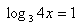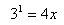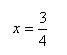Method 1

There are many different types of logarithmic equations. The first type uses properties to convert more than one log into a single log expression and then applies inverses to solve.

Example 1 Solve log3 4 + log3 x = 1

Step 1. Use properties to convert to a single log.Step 2. Translate the log equation to its equivalent exponential form.Step 3. Solve for x.